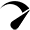# Solar DC To AC Conversion Calculator & Formula (Watts, Amps)

Use our solar DC to AC conversion calculator to convert the DC (direct current) power into usable AC (alternating current) power.

Direct current (DC) is the form of power produced by the solar panels and batteries are designed to store DC current (12v, 24v, 48v).

But most of our household appliances are designed to be run on Alternating current – AC (120-240V). So there will be a power loss when converting DC into AC with the help of an inverter.

Use the calculator below to figure out how many DC watts will be equal to AC watts and also keep reading for more in-depth knowledge about this topic if you’re a newbie in the solar world.

Two columns
Vertical
Horizontal

## Summary

{{ field.label }} {{ field.converted }} {{ field.extraView }}
{{ option.label }} {{ option.converted }}
{{ item.label }} {{ item.data.converted }}

Issued on: {{ \$store.getters.getIssuedOn }}
Payment method: {{ \$store.getters.getPaymentType }}
{{ item.label }}: {{ item.converted }}

Note: 1000Wh = 1kWh and most inverters are about 90% efficient. But to check the exact value, have a look at the specs of your inverter.

### Assumption

• There’ll be no power loss in AC wiring (use the chart below to figure out the right size wiring for your inverter).

### How To Use This Calculator?

DC watts: These can be the watts produced by your solar panels or the total capacity of your battery in Watts-hours to figure out how much actual power you have stored to run your household appliances.

Inverter Efficiency: Read the product description or specs sheet on your inverter (usually located at the bottom side). it’ll be mentioned as inverter efficiency rate (e.g. 90%). Then enter 90 in the calculator.

### Example

like I have two 200W portable solar panels that produce about 1500 watts of total power in a day (1500Wh) and I have a 1000-watt pure sine wave inverter (with 90% efficiency rate) to run my AC appliances.

### DC To AC Conversion Formula

Direct current (DC) is the form of power produced by the solar panels and batteries are designed to store DC current (12v, 24v, 48v)

But most of our household appliances are designed to be run on Alternating current – AC (120-240V). So there will be a power loss when converting DC into AC  with the help of an inverter.

To calculate the DC watts into AC watts use this formula:

Formula: Solar AC Watts = (DC watts × Inverter inverter efficiency rate) ÷ 100

Calculate DC amps into AC amps using this formula:

Formula: Solar AC amps = (DC amps × Inverter inverter efficiency rate) ÷ 100

## DC To AC Conversion Loss

When converting DC watts into AC watts there will be a conversion loss of 5-15% because of the inverter efficiency rate.

Internal temperature and standby power consumption of an inverter is the reason for their less efficient rate.

Also using the wrong size wire will cause some power loss because every size wire is designed to handle a limited amount of current.

So make sure to use the correct size wiring between the battery bank to the inverter.

Use this chart to select the right size wire from the battery bank for your inverter.

## DC To AC Conversion – Chart

Here’s a chart of DC watts into AC watts conversion with a pure sine wave inverter and modified sine wave inverter.

Note: The above table is based on a 90% efficient inverter.

## How Do I Convert DC Watts To AC Watts?

AdvertisementsTo calculate DC watts into AC watts multiply the DC watts by the inverter efficiency rate and divide the result by 100. For example, most inverters are 90% efficient.

So, (100 DC watts × 90) ÷ 100 = 90 AC watts.

With the help of this simple calculation formula, you can easily calculate the DC watts of your battery bank or solar panels into AC watts.

Scroll to TopOptimized by Seraphinite Accelerator
Turns on site high speed to be attractive for people and search engines.